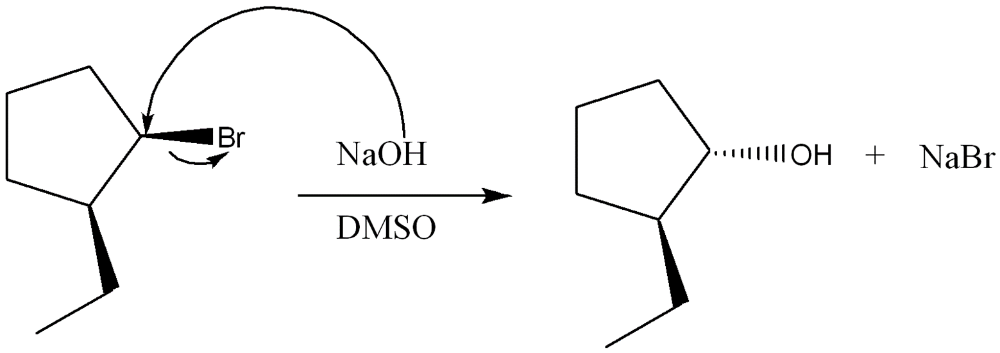# Chapter 7 - Alkyl Halides and Nucleophillic Substitution Flashcards

Set Details Share
created 2 years ago by alexisho1
290 views
updated 2 years ago by alexisho1
Page to share:
Embed this setcancel
COPY
code changes based on your size selection
Size:
X

1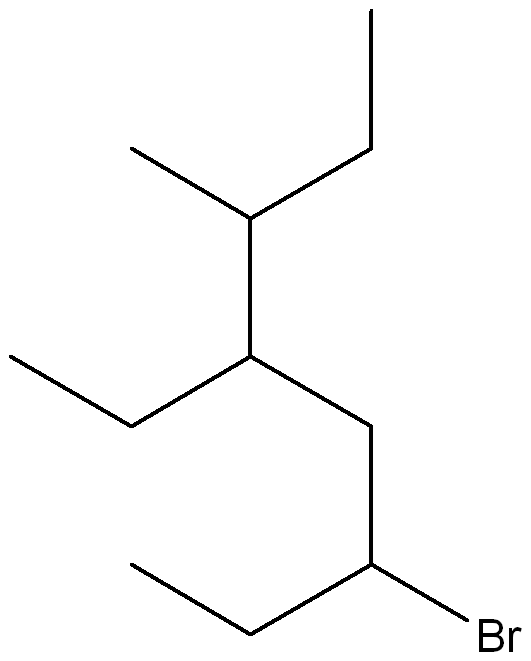Give the IUPAC name for the following compound

6-bromo-4-ethyl-3-methyloctane

2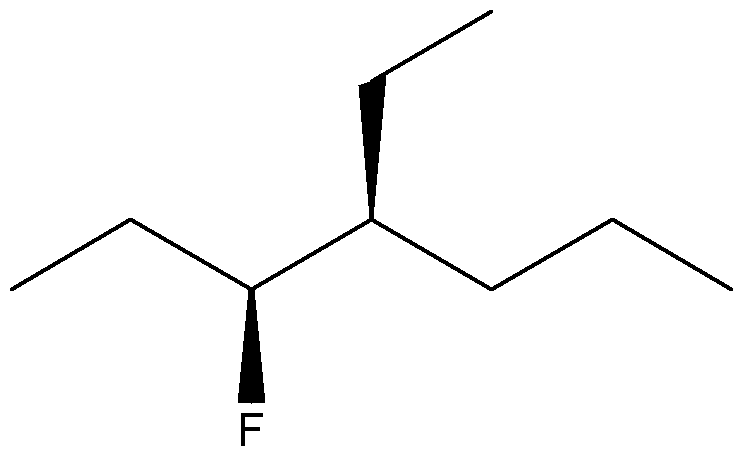Give the IUPAC name for the following compound

(3S, 4S)-4-ethyl-3-fluoroheptane

3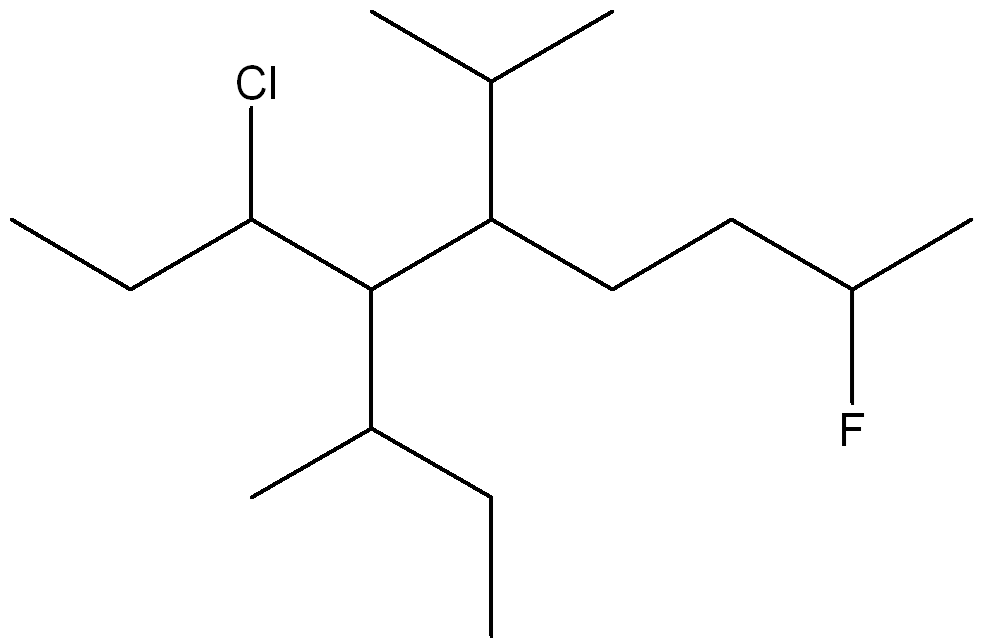Give the IUPAC name for the following compound

6-chloro-2-fluoro-5-isobutyl-7-methylnonane

4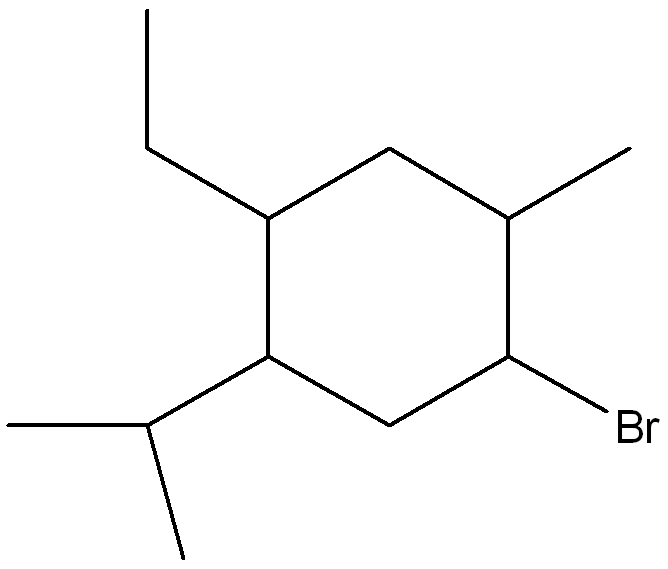Give the structure corresponding to the IUPAC Name giving

1-bromo-4-ethyl-5-isopropyl-2-methylcyclohexane

5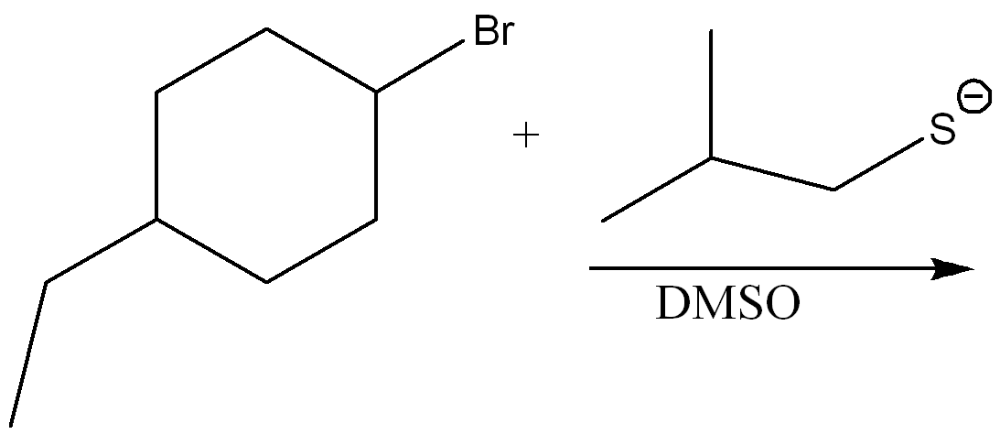Draw the products of the substitution reaction. Indicate the stereochemistry where appropriate.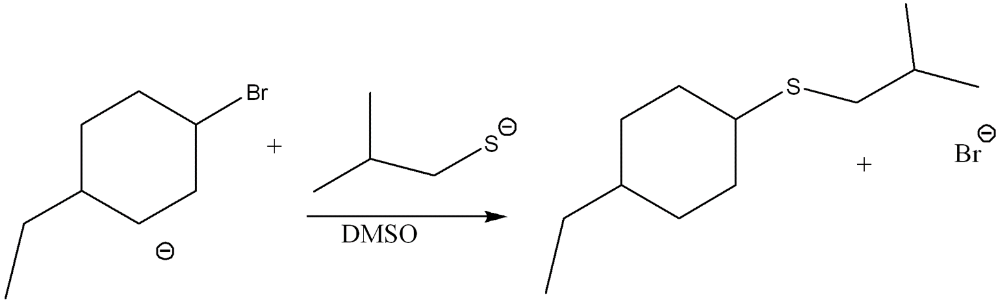6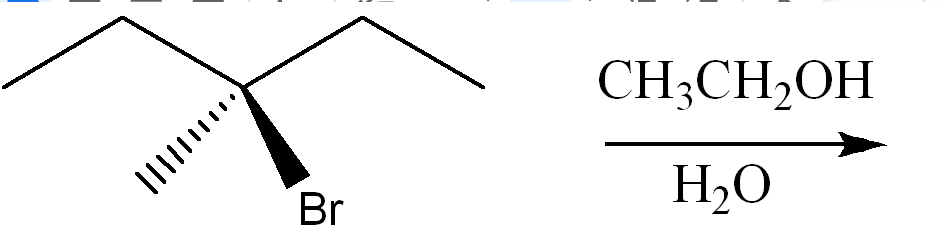Draw the products of the substitution reaction. Indicate the stereochemistry where appropriate.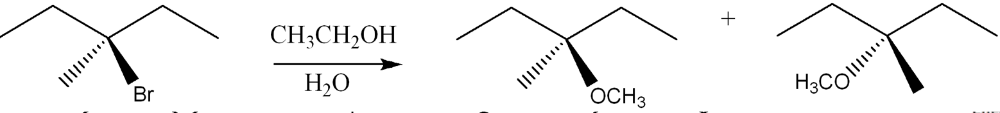7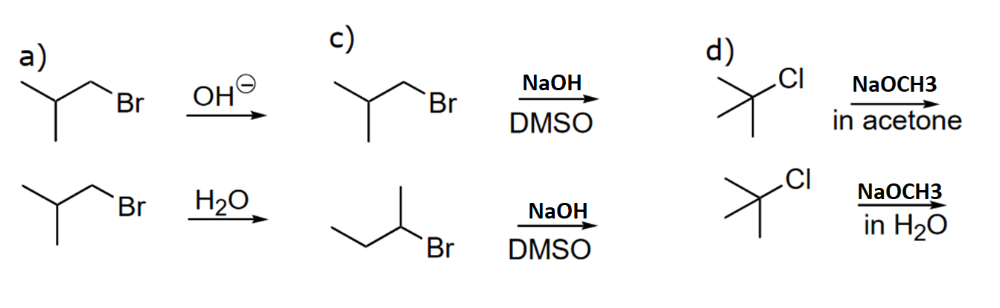Determine the faster substitution reaction among the following pairs and explain why?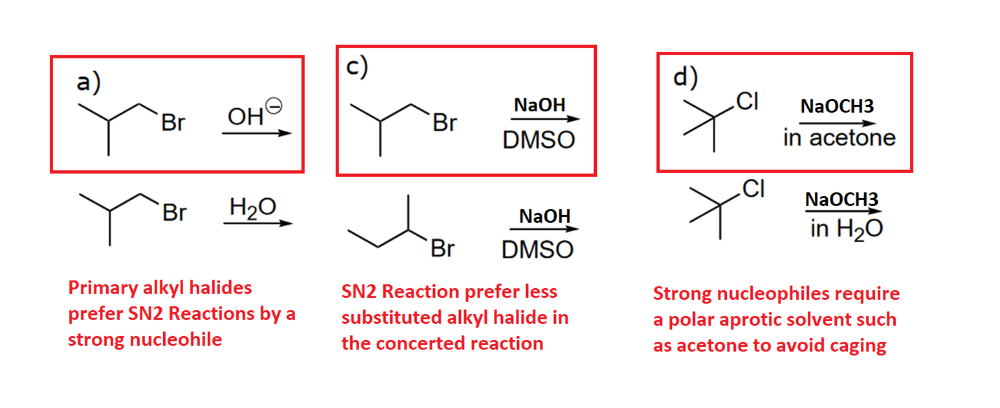8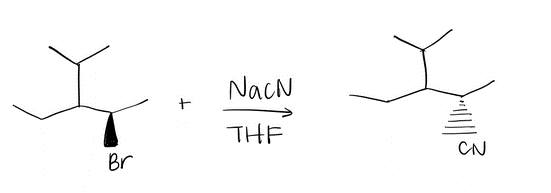Draw a mechanism using curved arrows. Draw an energy diagram including the labeled axis, reactants, products, Ea, and overall enthalpy. Assume the reaction is exothermic. Draw the structure of the transition state.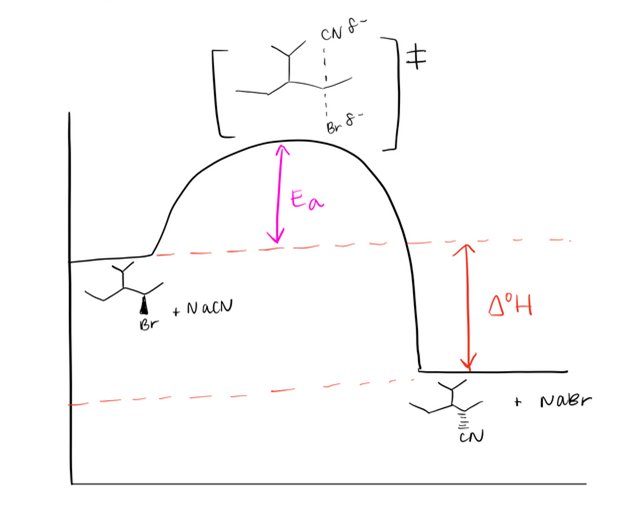9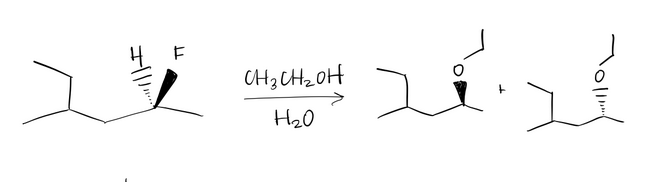Draw a mechanism using curved arrows. Draw an energy diagram including the labeled axis, reactants, products, Ea, and overall enthalpy. Assume the reaction is endothermic. Draw the structure of the transition state.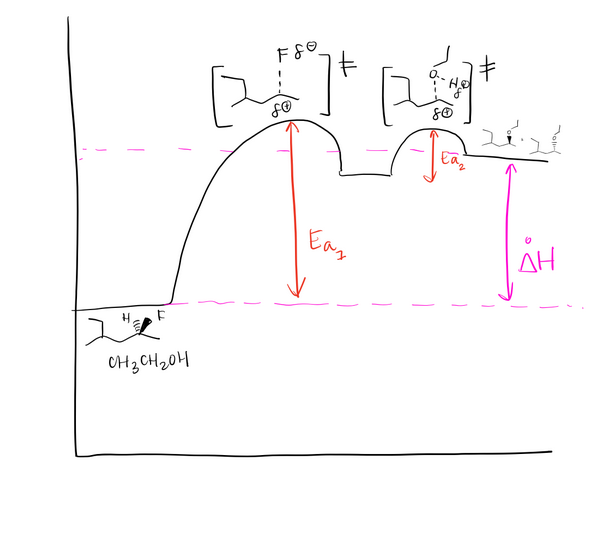10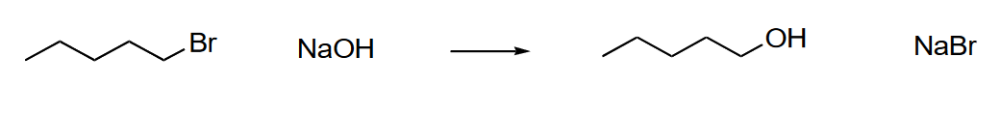What is the rate equation for the following reaction.

A. What happens if the leaving group is changed from Br to F

B. What happens if the solvent is changed from CH3OH and DMSO

C. The concentration of Sodium Hydroxide is increased by a factor of 2

D. The concentration of the Alkyl Halide and Sodium Hydroxide is decreased by a factor of 5

Rate = [Alkyl Halide] [-OH]

A. Rate decreased

B. Rate increased

C. Rate increased by a factor of 2

D. Rate is decreased by a factor of 25

11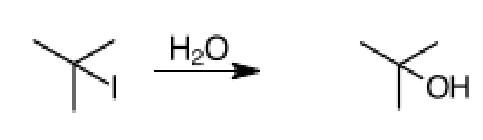What is the rate equation for the following reaction.

A. What happens if the leaving group is changed to I to Cl

B. What happens if the solvent is changed from THF to CH3OH

C. The concentration of water is tripled

D. The concentration of water and the alkyl halide is decreased by a factor of 4

Rate = [Alkyl Halide]

A. Rate decreased

B. Rate increased

C. The rate does not change

C. Rate is decreased by a factor of 4

12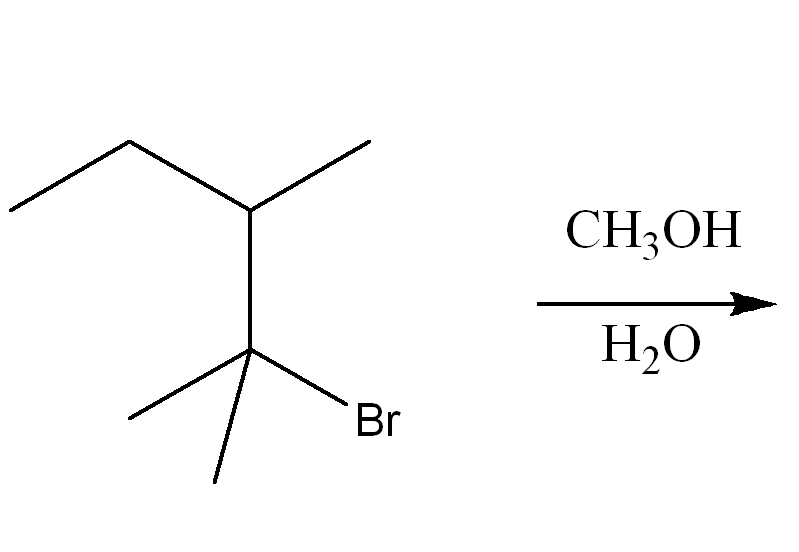Determine the mechanism of nucleophilic substitution of the reaction using curved arrow notation. Draw the products, including stereochemistry if applicable.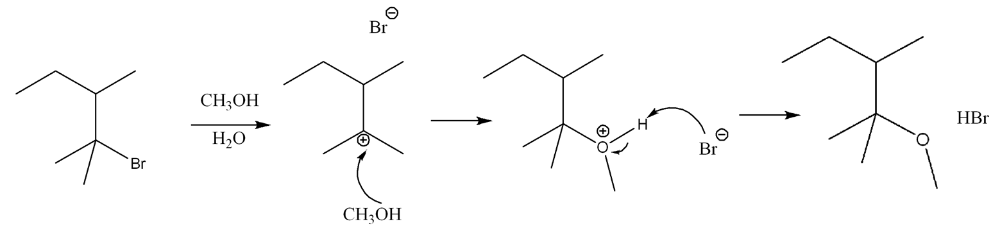13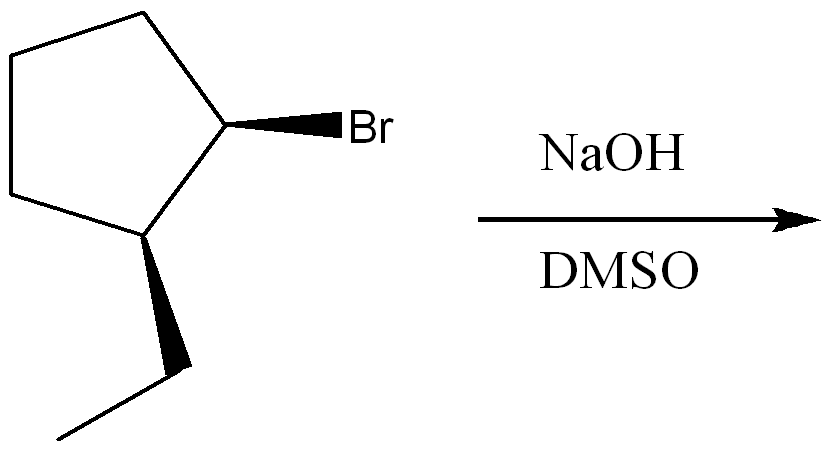Determine the mechanism of nucleophilic substitution of the reaction using curved arrow notation. Draw the products, including stereochemistry.